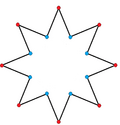Kids Encyclopedia Facts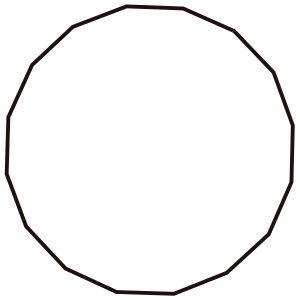Type Regular polygon
Edges and vertices 16
Schläfli symbol {16}, t{8}, tt{4}
Coxeter diagram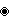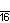Symmetry group Dihedral (D16), order 2×16
Internal angle (degrees) 157.5°
Dual polygon Self
Properties Convex, cyclic, equilateral, isogonal, isotoxal

An hexadecagon or hekkaidecagon is a shape with 16 sides and 16 corners.

## Contents

A regular hexadecagon is a hexadecagon in which all angles are equal and all sides are congruent. Its Schläfli symbol is {16} and can be constructed as a truncated octagon, t{8}, and a twice-truncated square tt{4}. A truncated hexadecagon, t{16}, is a triacontadigon, {32}.

### Area

The area of a regular hexadecagon with edge length t is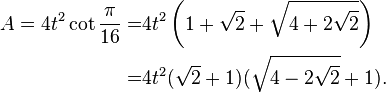\begin{align} A = 4t^2 \cot \frac{\pi}{16} =& 4t^2 \left(1+\sqrt{2}+\sqrt{ 4+2\sqrt{2} }\right)\\ =& 4t^2 (\sqrt{2}+1)(\sqrt{4-2\sqrt{2}}+1) .\end{align}

Because the hexadecagon has a number of sides that is a power of two, its area can be computed in terms of the circumradius R by truncating Viète's formula: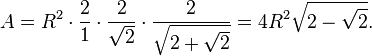$A=R^2\cdot\frac{2}{1}\cdot\frac{2}{\sqrt{2}}\cdot\frac{2}{\sqrt{2+\sqrt{2}}}=4R^2\sqrt{2-\sqrt{2}}.$

Since the area of the circumcircle is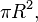$\pi R^2,$ the regular hexadecagon fills approximately 97.45% of its circumcircle.

## Dissection

Coxeter states that every parallel-sided 2m-gon can be divided into m(m-1)/2 rhombs. For the regular hexadecagon, m=8, and it can be divided into 28: 4 squares and 3 sets of 8 rhombs. This decomposition is based on a Petrie polygon projection of a 8-cube, with 28 of 1792 faces. The list A006245 enumerates the number of solutions as 1232944, including up to 16-fold rotations and chiral forms in reflection.

{8}#{ } { 83 }#{ } { 85 }#{ }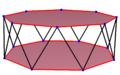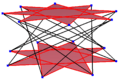A regular skew hexadecagon is seen as zig-zagging edges of a octagonal antiprism, a octagrammic antiprism, and a octagrammic crossed-antiprism.

A skew hexadecagon is a skew polygon with 24 vertices and edges but not existing on the same plane. The interior of such an hexadecagon is not generally defined. A skew zig-zag hexadecagon has vertices alternating between two parallel planes.

A regular skew hexadecagon is vertex-transitive with equal edge lengths. In 3-dimensions it will be a zig-zag skew hexadecagon and can be seen in the vertices and side edges of a octagonal antiprism with the same D8d, [2+,16] symmetry, order 32. The octagrammic antiprism, s{2,16/3} and octagrammic crossed-antiprism, s{2,16/5} also have regular skew octagons.

## In art

In the early 16th century, Raphael was the first to construct a perspective image of a regular hexadecagon: the tower in his painting The Marriage of the Virgin has 16 sides, elaborating on an eight-sided tower in a previous painting by Pietro Perugino.

Hexadecagrams (16-sided star polygons) are included in the Girih patterns in the Alhambra.